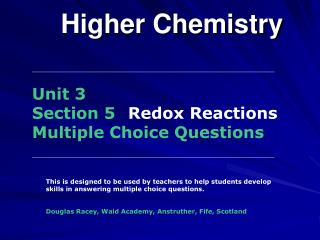Download PresentationHigher ChemistryHigher Chemistry - PowerPoint PPT Presentation

Download PresentationHigher Chemistry
An Image/Link below is provided (as is) to download presentation

Download Policy: Content on the Website is provided to you AS IS for your information and personal use and may not be sold / licensed / shared on other websites without getting consent from its author. While downloading, if for some reason you are not able to download a presentation, the publisher may have deleted the file from their server.

- - - - - - - - - - - - - - - - - - - - - - - - - - - E N D - - - - - - - - - - - - - - - - - - - - - - - - - - -
Presentation Transcript

1. Higher Chemistry Unit 3Section 5 Redox ReactionsMultiple Choice Questions This is designed to be used by teachers to help students develop skills in answering multiple choice questions. Douglas Racey, Waid Academy, Anstruther, Fife, Scotland

2. Higher Chemistry Unit 3 - Section 5 Redox ReactionsMultiple Choice Questions 1. If 96,500C of electricity are passed through separate solutions of copper(II)chloride and nickel(II) chloride, then A. equal masses of copper and nickel will be deposited B. the same number of atoms of each metal will be deposited C. the metals will be plated on the positive electrode D. different numbers of moles of each metal will be deposited B. Answer

3. Higher Chemistry Unit 3 - Section 5 Redox ReactionsMultiple Choice Questions 2. The ion-electron equations for a redox reaction are 2I-(aq)  I2(aq) + 2e- MnO4-(aq) + 8H+(aq) + 5e- Mn2+(aq) + 4H2O(l) How many moles of iodide ions are oxidised by one mole of permanganate ions? A. 0.2 B. 0.4 C. 2 D. 5 D. Answer

4. Higher Chemistry Unit 3 - Section 5 Redox ReactionsMultiple Choice Questions 3. In which of the following reactions is hydrogen gas acting as an oxidising agent? A. H2 + C2H4 C2H6 B. H2 + Cl2 2HCl C. H2 + 2Na  2NaH D. H2 + CuO  H2O + Cu D. Answer

5. Higher Chemistry Unit 3 - Section 5 Redox ReactionsMultiple Choice Questions 4. Which of the following is a redox reaction? A. NaOH + HCl  NaCl + H2O B. Zn + 2HCl  ZnCl2 + H2 C. NiO + 2HCl  NiCl2 + H2O D. CuCO3 + 2HCl  CuCl2 + H2O + CO2 B. Answer

6. Higher Chemistry Unit 3 - Section 5 Redox ReactionsMultiple Choice Questions 5. The reduction of copper ions during electroplating can be represented as: Cu2+(aq) + 2e- Cu(s) What is the quantity of electricity needed to produce 0.25 moles of copper? A. 24 125 C B. 48 250 C C. 96 500 C D. 193 000 C B. Answer

7. Higher Chemistry Unit 3 - Section 5 Redox ReactionsMultiple Choice Questions 6. Which of the following is a redox reaction? A. Mg + 2HCl  MgCl2 + H2 B. MgCO3 + 2HCl  MgCl2 + H2O+ CO2 C. MgO + 2HCl  MgCl2 + H2O D. Mg(OH) 2+ 2HCl  MgCl2 + H2O A. Answer

8. Higher Chemistry Unit 3 - Section 5 Redox ReactionsMultiple Choice Questions 7. The iodate ion, IO3-, can be converted to iodine. Which is the correct ion-electron equation for the reaction? A. 2IO3- + 12H+ +12e- 2I- + 6H2O B. IO3- + 6H+ +7e- I- + 3H2O C. 2IO3- + 12H+ +11e- I2 + 6H2O D. 2IO3- + 12H+ +10e- I2 + 6H2O D. Answer

9. Higher Chemistry Unit 3 - Section 5 Redox ReactionsMultiple Choice Questions 8. HgCl2(aq) + SnCl2 (aq)  Hg(l) + SnCl4(aq) What ion is oxidised in the above redox reaction? A. Sn2+(aq) B. Sn4+(aq) C. Hg2+(aq) D. Cl-(aq) A. Answer

10. Higher Chemistry Unit 3 - Section 5 Redox ReactionsMultiple Choice Questions 9. HgCl2(aq) + SnCl2 (aq)  Hg(l) + SnCl4(aq) What ion is the oxidising agent in the above redox reaction? A. Sn2+(aq) B. Sn4+(aq) C. Hg2+(aq) D. Cl-(aq) C. Answer

11. Higher Chemistry Unit 3 - Section 5 Redox ReactionsMultiple Choice Questions 10. The ion-electron equations for a redox reaction are 2I-(aq)  I2(aq) + 2e- MnO4-(aq) + 8H+(aq) + 5e- Mn2+(aq) + 4H2O(l) How many moles of iodide ions are oxidised by one mole of permanganate ions? A. 0.2 B. 0.4 C. 2 D. 5 D. Answer